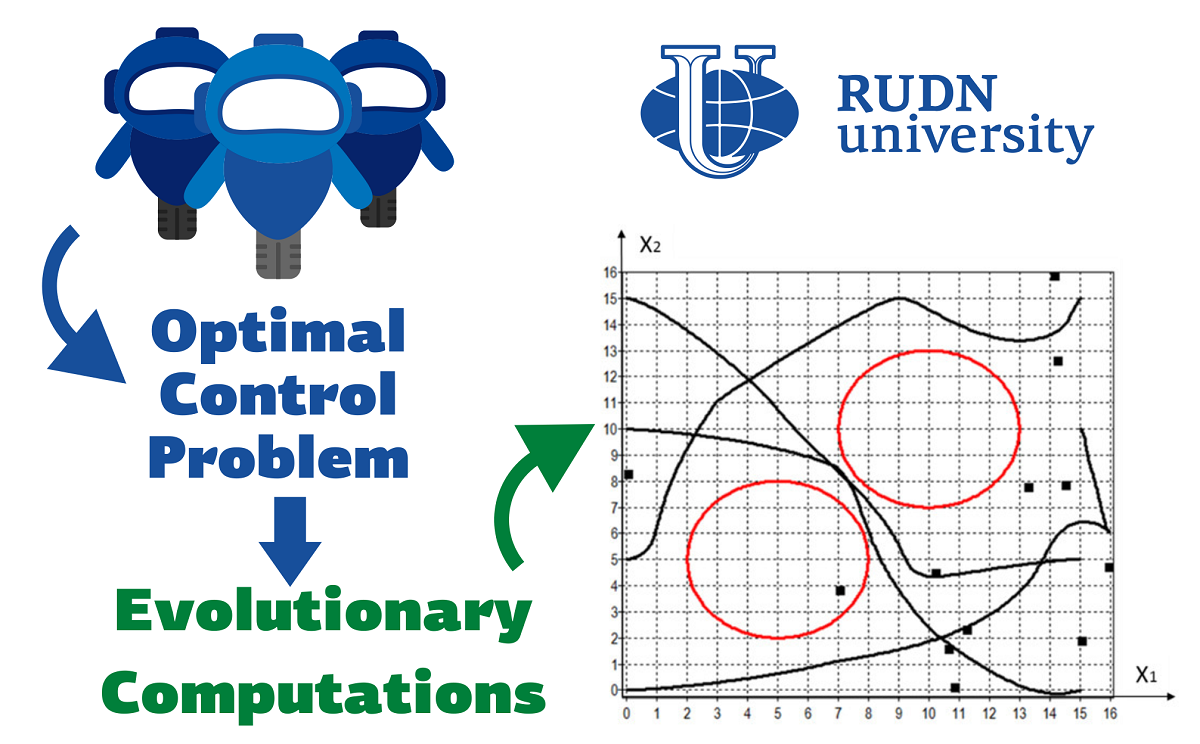RUDN University scientist compares algorithms for solving the optimal control problem

# RUDN University scientist compares algorithms for solving the optimal control problem

The RUDN University scientist compared the performance of several algorithms for solving optimal control problems that arise everywhere, from economics to cosmonautics.

Systems of several objects with an assigned sequence of actions are described with so-called optimal control problem. They arise for example, in controlling a spaceship or managing a country’s tax system. Mathematically, this means that one needs to minimize or maximize some parameter of the system (for example, minimize time or maximize employment). There is no generally accepted universal way to analyse such systems numerically, but there are many approaches and algorithms. Researchers from RUDN University and Federal Research Center “Computer Science and Control” of the Russian Academy of Sciences have proposed two approaches based on several modern computer algorithms for solving the problem of optimal control of a group of robots.

“A group of robots should move from given initial states to terminal ones while avoiding obstacles in a minimum time. The problem belongs to the class of infinite-dimensional optimization. There are two approaches to solve it numerically. A direct approach is based on a discretization of the control function and reduction to the finite-dimensional optimization. An indirect approach is based on the Pontryagin maximum principle for the transition to the boundary value problem and its numerical solution”, said Sergey Konstantinov, Senior Lecturer of the на Department of Mechanics and Control Processes of RUDN University.

Scientists have proposed two approaches to solving the optimal control problem based on direct methods. In a test, robots should move from the starting point to the end point and not collide with obstacles and other robots. In the first approach, a group of robots was considered as a single object. In this case, the optimal control problem is reduced to a non-linear programming problem. This means that it cannot be reduced to a system of linear equations, which complicates the problem. In the second approach, they first find attractors for each robot — special points on the plane, that “tell” the robot how to avoid obstacles on the way. The results obtained were then used to solve the entire original problem. Calculations based on two approaches were implemented using evolutionary algorithms and the random search method. The researchers conducted 10 tests for each of the four evolutionary algorithms and the random search method and compared their performance.

The effectiveness of two approaches and 5 algorithms (the random search method and 4 evolutionary algorithms: the genetic algorithm, particle swarm optimization, bee algorithm, and gray wolf optimizer) was evaluated based on the value of the objective function — the function that needs to be minimized in the optimal control problem. The smaller it is, the better the algorithm performed. For the first approach, all evolutionary algorithms turned out to be more efficient than the random search method. The particle swarm optimization performed best, with an average value of 5.5 for the objective function. For the random search method, this value was almost three times higher — 15.83. For the second approach, the random search method also proved to be the least effective. The evolutionary algorithms worked about equally efficiently. In one of the tests, gray wolf optimizer gave the minimum value of the objective function — 2.49.

“Currently, there are no universal numerical methods for solving optimal control problems. We plan to continue the study of evolutionary algorithms and consider other new evolutionary algorithms, including hybrid ones”, said Sergey Konstantinov, Senior Lecturer of the Department of Mechanics and Mechatronics of RUDN University.

The results are published in the journal Applied Sciences.News
All news
Science
20 Sep
RUDN University mathematician determined the conditions for the coexistence of three species in the wild

RUDN University mathematician together with colleagues from India and France for the first time studied in detail the system of coexistence of three species of living creatures in the wild. The results help to understand what parameters determine the extinction andли survivalof species, and how the number of species changes in space and time.

Science
15 Sep
RUDN University Chemist Created Coordination Polymers Films with up to 99.99% antibacterial efficiency

RUDN University chemist with his colleagues from Portugal has developed two types of coating based on new coordination polymers with silver. Both compounds were successfully tested against four common pathogens.

Science
12 Sep
RUDN scientists have improved titanium dental implants with graphene nanosloyers

RUDN researchers have created and tested a method for processing titanium dental implants. It turned out that theanoslos of graphene on the surface of titanium improve its interaction with stem cells,which are placed on the implant so that it better "takes root".Thanks to this method ofprocessing, stem cells are better kept on the surface, multiply and turn into the desired cells.